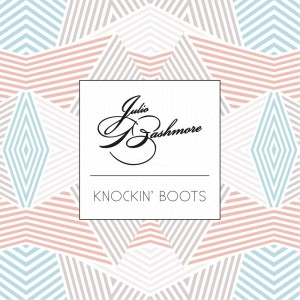# Simple Love

7 Aug 2015

## Simple Love Lyrics

Simple Love (简单爱) - Julio Bashmore/J'Danna

This would be so simple

If only you would love

This would be so simple

If only you would love

This would be so simple

If only you would love

This would be so simple

This would be

This would be so simple

This would be so simple

This would be

This would be so simple

If only you would love

This would be so simple

If only you would love

This would be so simple

If only you would love

This would be so simple

If only you would love

This would be so simple

If only you would love

This would be so simple

This would be

This would be so simple

This would be so simple

This would be

This would be so simple

If only you would love